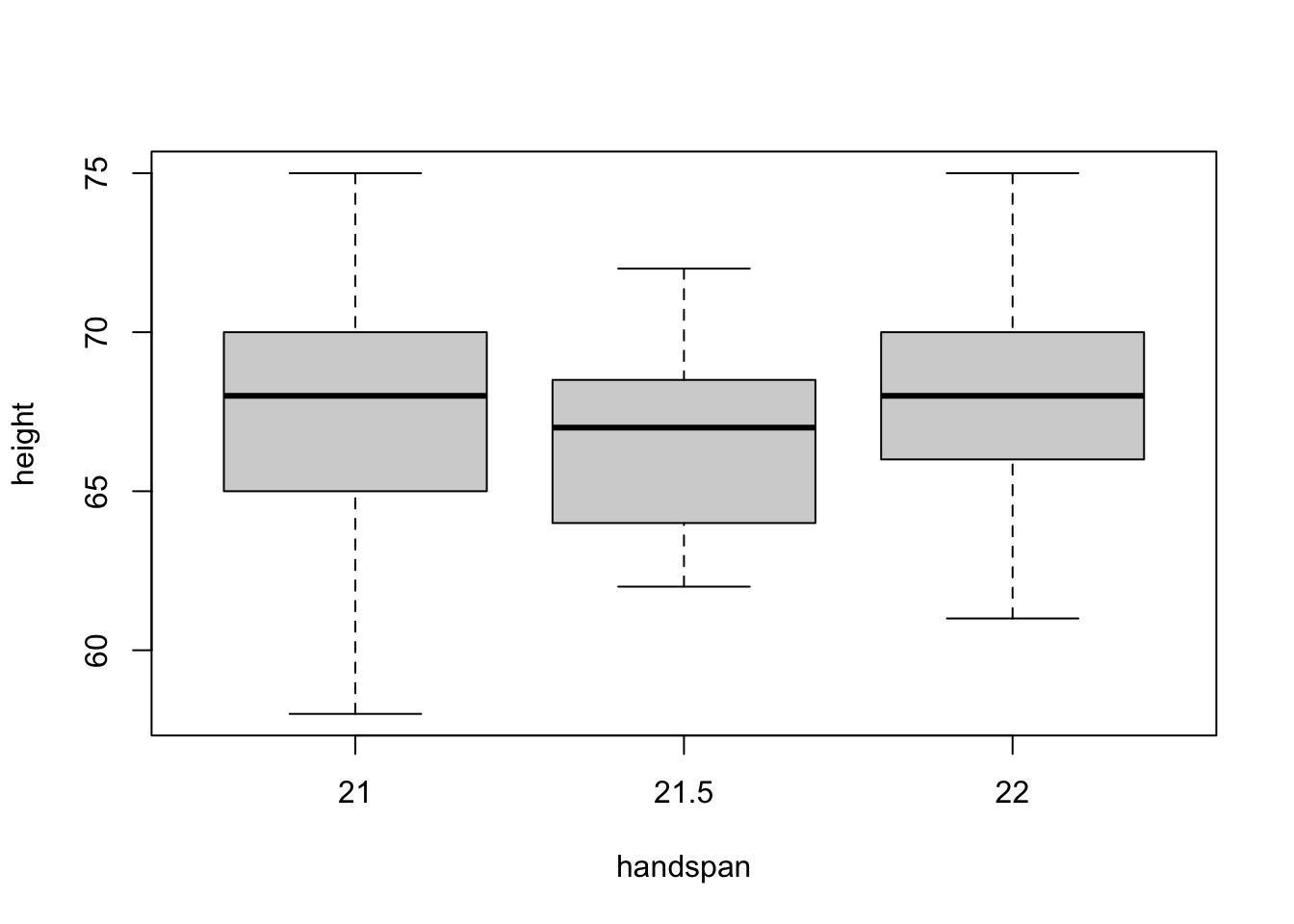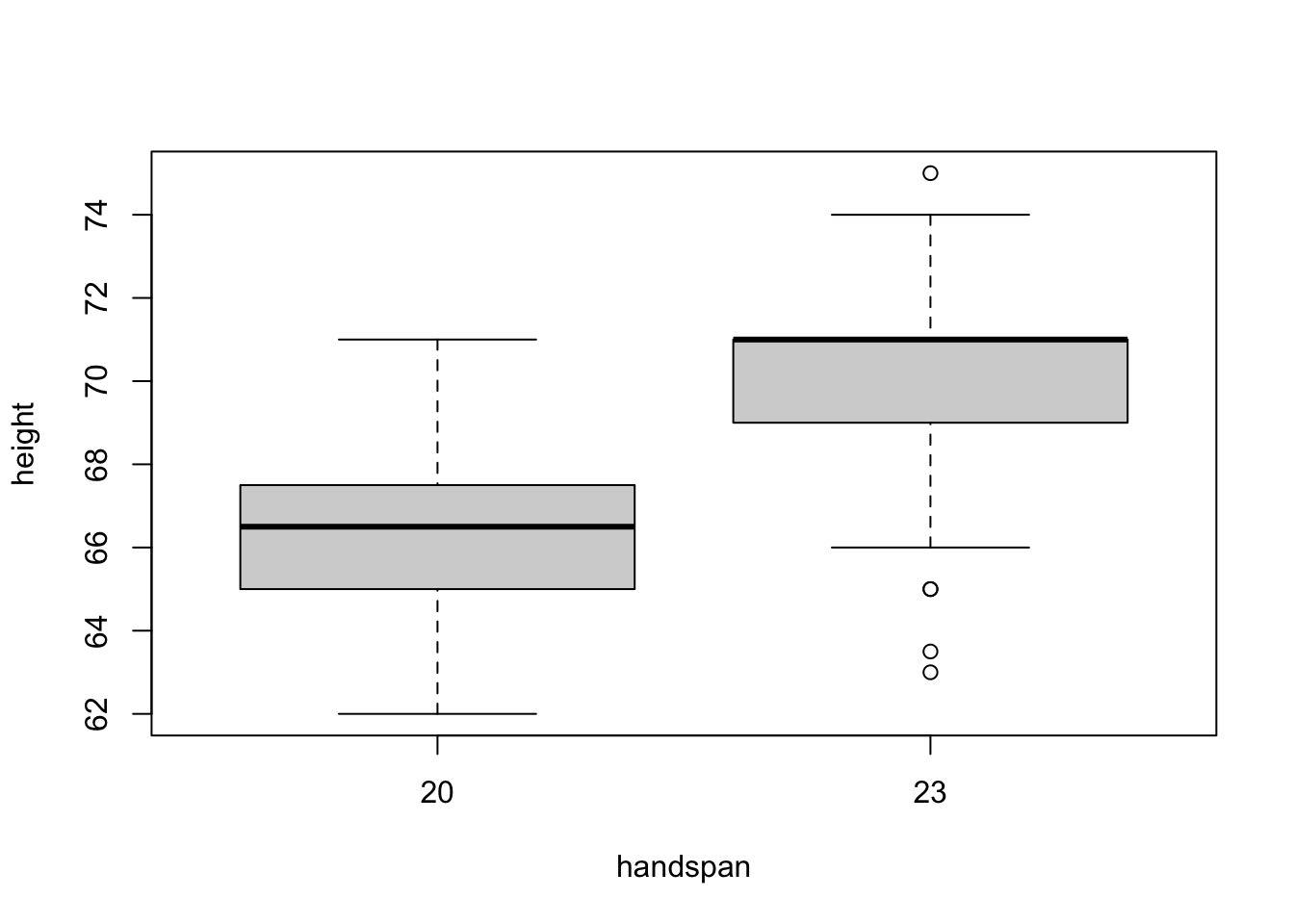# A New Way of Looking at Least Squares

Have you got a ruler handy? Fantastic! Then hold out your right hand, extend your thumb and little finger as far as they’ll go, and measure the distance in centimeters, rounding to the nearest half centimeter. This is your handspan. Mine is around 23.5 centimeters, but is that big, small, or merely average?1 Fortunately for you, I’ve asked hundreds of introductory statistics students to measure their handspans over the years and (with their consent) posted the resulting data on my website:

library(tidyverse)
make_pairs(dat$height)) head(regression_pairs) ## X1 X2 X1.1 X2.1 ## 1 22.5 17.0 73 65 ## 2 22.5 21.0 73 69 ## 3 22.5 25.5 73 71 ## 4 22.5 25.0 73 78 ## 5 22.5 20.0 73 68 ## 6 22.5 20.5 73 75 The names are ugly, so let’s clean them up a bit. Handspan is our “x” variable and height is our “y” variable, so we’ll refer to the measurements from each pair as x1, x2, y1, y2 names(regression_pairs) <- c('x1', 'x2', 'y1', 'y2') head(regression_pairs) ## x1 x2 y1 y2 ## 1 22.5 17.0 73 65 ## 2 22.5 21.0 73 69 ## 3 22.5 25.5 73 71 ## 4 22.5 25.0 73 78 ## 5 22.5 20.0 73 68 ## 6 22.5 20.5 73 75 The 1 and 2 indices indicate a particular student: in a given row x1 and y1 are the handspan and height of the “first student” in the pair while x2 and y2 are the handspan and height of the “second student.” Each student appears many times in the regression_pairs dataframe. This is because there are many pairs of students that include Alice: we can pair her up with any other student in the class. For this reason, regression_pairs has a tremendous number of rows, 52975 to be precise. This is the number of ways to form a committee of size 2 from a collection of 326 people when order doesn’t matter: choose(326, 2) ##  52975 # I just calculated 52,975 slopes. Corresponding to each row of regression_pairs is a slope. We can calculate and summarize them as follows, using the dplyr package from the tidyverse to make things easier to read: library(dplyr) regression_pairs <- regression_pairs %>% mutate(slope = (y2 - y1) / (x2 - x1)) regression_pairs %>% pull(slope) %>% summary ## Min. 1st Qu. Median Mean 3rd Qu. Max. NA's ## -Inf 0.000 1.333 NaN 2.667 Inf 419 The sample mean slope is NaN, the minimum is -Inf, the maximum is Inf, and there are 419 missing values. So what on earth does this mean? First things first: the abbreviation NaN stands for “not a number.” This is R’s way of expressing $$0/0$$, and an NaN “counts” as a missing value: 0/0 ##  NaN is.na(0/0) ##  TRUE In contrast, Inf and -Inf are R’s way of expressing $$\pm \infty$$. These do not count as missing values, and they also arise when a number is too big or too small for your computer to represent: c(-1/0, 1/0, exp(9999999), -exp(9999999)) ##  -Inf Inf Inf -Inf is.na(c(Inf, -Inf)) ##  FALSE FALSE So where do these Inf and NaN values come from? Our slope calculation from above was (y2 - y1) / (x2 - x1). If x2 == x1, the denominator is zero. This occurs when the two students in a given pair have the same handspan. Because we only measured handspan to the nearest 0.5cm, there are many such pairs. Indeed, handspan only takes on 23 distinct values in our dataset but there are 326 students: sort(unique(dat$handspan))
##   16.5 17.0 17.5 18.0 18.5 19.0 19.5 20.0 20.5 21.0 21.5 22.0 22.5 23.0 23.5
##  24.0 24.5 25.0 25.5 26.0 26.5 27.0 28.0

If y1 and y2 are different but x1 and x2 are the same, the slope will either be Inf or -Inf, depending on whether y1 > y2 or the reverse. When y1 == y2 and x1 == x2 the slope is NaN.

This isn’t an arcane numerical problem. When y1 == y2 and x1 == x2, our pair contains only a single point, so there’s no way to draw a line segment. With no line to draw, there’s clearly no slope to calculate. When y1 != y2 but x1 == x2 we can draw a line segment, but it will be vertical. Should we call the slope of this vertical line $$+\infty$$? Or should we call it $$-\infty$$? Because the labels 1 and 2 for the students in a given pair were arbitrary–order doesn’t matter–there’s no way to choose between Inf and -Inf. From the perspective of calculating a slope, it simply doesn’t make sense to construct pairs of students with the same handspan.

With this in mind, let’s see what happens if we average all of the slopes that are not -Inf, Inf, or NaN

regression_pairs %>%
filter(!is.na(slope) & !is.infinite(slope)) %>%
pull(slope) %>%
mean
##  1.259927

This is tantalizingly close to the slope of the regression line from above: 1.266961. But it’s still slightly off.

# Not All Slopes are Created Equal

The median handspan in my dataset is 21.5. Let’s take a closer look at the heights of students whose handspans are close to this value:

boxplot(height ~ handspan, dat, subset = handspan %in% c(21, 21.5, 22))There is a large amount of variation in height for a given value of handspan. Indeed, from this boxplot alone you might not even guess that there is a strong positive relationship between height and handspan in the dataset as a whole! The “boxes” in the figure, representing the middle 50% of heights for a given handspan, overlap substantially. If we were to randomly choose one student with a handspan of 21 and another with a handspan of 21.5, it’s quite likely that the slope between them would be negative. It’s true that students with bigger hands are taller on average. But the difference in height that we’d predict for two students who differed by 0.5cm in handspan is very small: 0.6 inches according to the linear regression from the beginning of this post. In contrast, the standard deviation of height among students with the median handspan is more than five times as large:

sd(subset(dat, handspan == 21.5)\$height)
##  3.172248

The 25th percentile of handspan is 20 while the 75th percentile is 23. Comparing the heights of students with these handspans rather than those close to the median, gives a very different picture:

boxplot(height ~ handspan, dat, subset = handspan %in% c(20, 23))Now there’s much less overlap in the distributions of height. This accords with the predictions of the linear regression from above: for two students whose handspan differs by 3cm, we would predict a difference of 3.8 inches in height. This difference is big enough to discern in spite of the variation in height for students with the same handspan. If we were to choose one student with a handspan of 20cm and another with a handspan of 23cm, it’s fairly unlikely that the slope between these lines would be negative.

So where does this leave us? Above we saw that forming a pair of students with the same handspan does not allow us to calculate a slope. Now we’ve seen that the slope for a pair of students with a very similar handspan can give a misleading impression about the overall relationship. This turns out to be the key to our puzzle from above. The ordinary least squares slope estimate does equal an average of the slopes for each pair of students, but this average gives more weight to pairs with a larger difference in handspan. As I’ll derive in my next post: $\widehat{\beta}_{\text{OLS}} = \sum_{(i,j)\in C_2^n} \omega_{ij} \left(\frac{y_i - y_j}{x_i - x_j}\right), \quad \omega_{ij} \equiv \frac{(x_i - x_j)^2}{\sum_{(i,j)\in C_2^n} (x_i-x_j)^2}$ The notation $$C_2^n$$ is shorthand for the set $$\{(i,j)\colon 1 \leq i < j \leq n\}$$, in other words the set of all unique pairs $$(i,j)$$ where order doesn’t matter. The weights $$\omega_{ij}$$ are between zero and one and sum to one over all pairs. Pairs with $$x_i = x_j$$ are given zero weight; pairs in which $$x_i$$ is far from $$x_j$$ are given more weight than pairs where these values are closer. And you don’t have to wait for my next post to see that it works:

regression_pairs <- regression_pairs %>%
mutate(x_dist = abs(x2 - x1),
weight = x_dist^2 / sum(x_dist^2))

regression_pairs %>%
filter(!is.infinite(slope) & !is.na(slope)) %>%
summarize(sum(weight * slope)) %>%
pull
##  1.266961
coef(lm(height ~ handspan, dat))
## handspan
## 1.266961

So there you have it: in a simple linear regression, the OLS slope estimate is a weighted average of the slopes of the line segments between all pairs of observations. The weights are proportional to the squared Euclidean distance between $$x$$-coordinates. I’ll leave things here for today, but there’s much more to say on this topic. Stay tuned for the next installment!

1. If you play the piano, this may help: I can play parallel 10ths but only just.↩︎

2. Another way to show this is by “jittering” the data: simply replace geom_point(alpha = 0.3) with geom_jitter(alpha = 0.3).↩︎